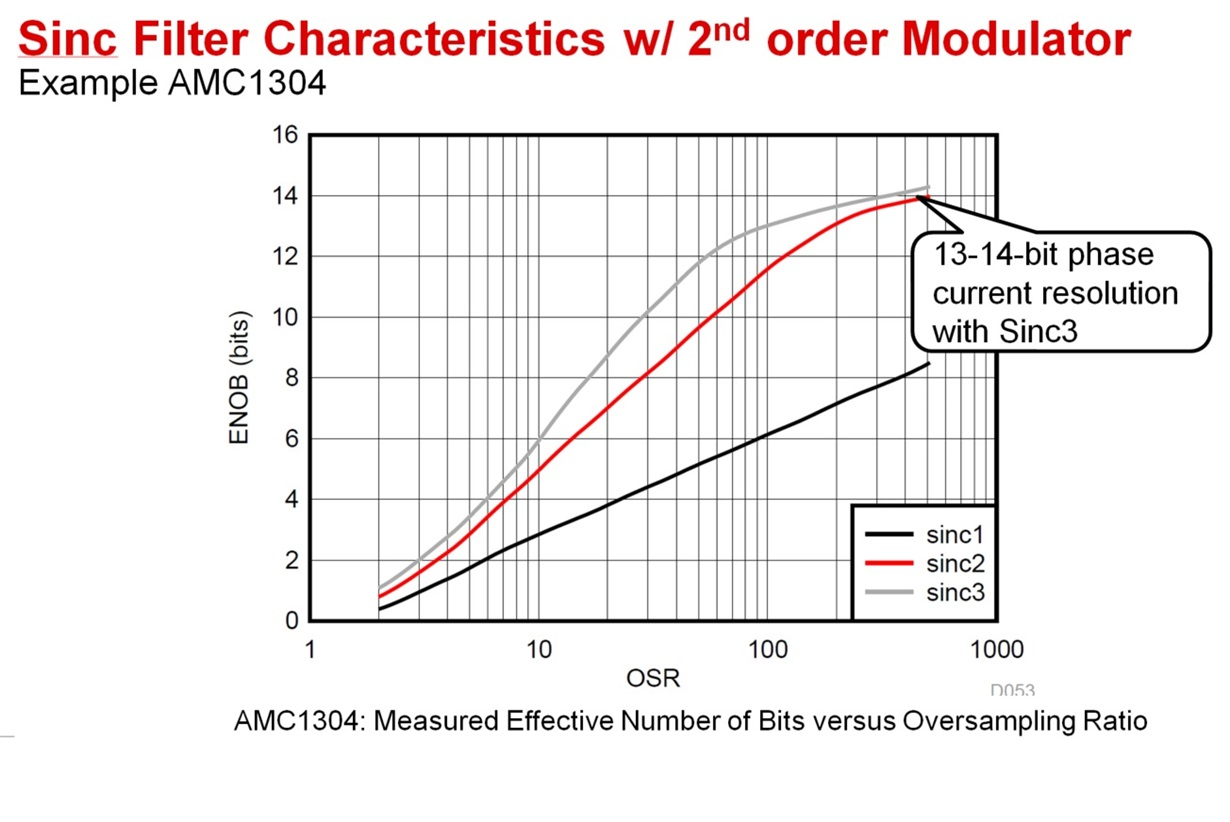If you have a related question, please click the "Ask a related question" button in the top right corner. The newly created question will be automatically linked to this question.

# AMC1304M05: Understanding the resolution of AMC1304 and sigma-delta devices

Part Number: AMC1304M05
Other Parts Discussed in Thread: AMC1210

Hi team,

I am helping customer to test AMC1304 together with C2000. Thus I am trying get some detailed knowledge on this devices.

When I am looking into the resolution of AMC1304, it makes me a little bit confused.

The question is about the following figure:Assuming we are using a OSR=10. For Sinc1 filter, it is easy to understand the max number of "1" sampled will be 10, thus the resolution will be ~3bit which matches the figure.

However, for OSR=10 Sinc2 filter,  according to my understanding, the max number we are getting is actually 100 (10*10) which brings a 6-7 bit resolution. And this does not match the figure.

Regards,

Brian

• Hi Brian,

Tell Luke I said hello!  Your understanding is correct for the max number, and I believe there is a graph in the C2000 datasheet similar to the one shown in the AMC1210 datasheet (Figure 16).  You are comparing this against ENOB though, which is not the same thing as the SINC filter resolution.

• Hi Tom,

So basically, you are suggesting that when OSR=10 the resolution of Sinc1 is about 3bit(10 samples), and Sinc2 is about 6-7 bit(100 samples) and Sinc3 is about 10-bit (1000 samples) theoretically. But due to the limitation of device, the ENOB can actually not meet that number?

Could you help to explain what brings such big gap between the theoretical resolution and ENOB?

Thanks and Regards,

Brian

• Hi Brian,

In a nutshell, it's because of noise.  ENOB is derived from the SNR of an ADC.  Peggy does a great job explaining SNR in the TI Precision Labs - ADC series, section 1.1.  The theoretical dynamic range of an N-bit ADC is based on its signal-to-noise ratio, where SNR = 1.76 + 6.02N dB.  You can rearrange the equation to get ENOB where N effective = (SNR - 1.76) / 6.02.